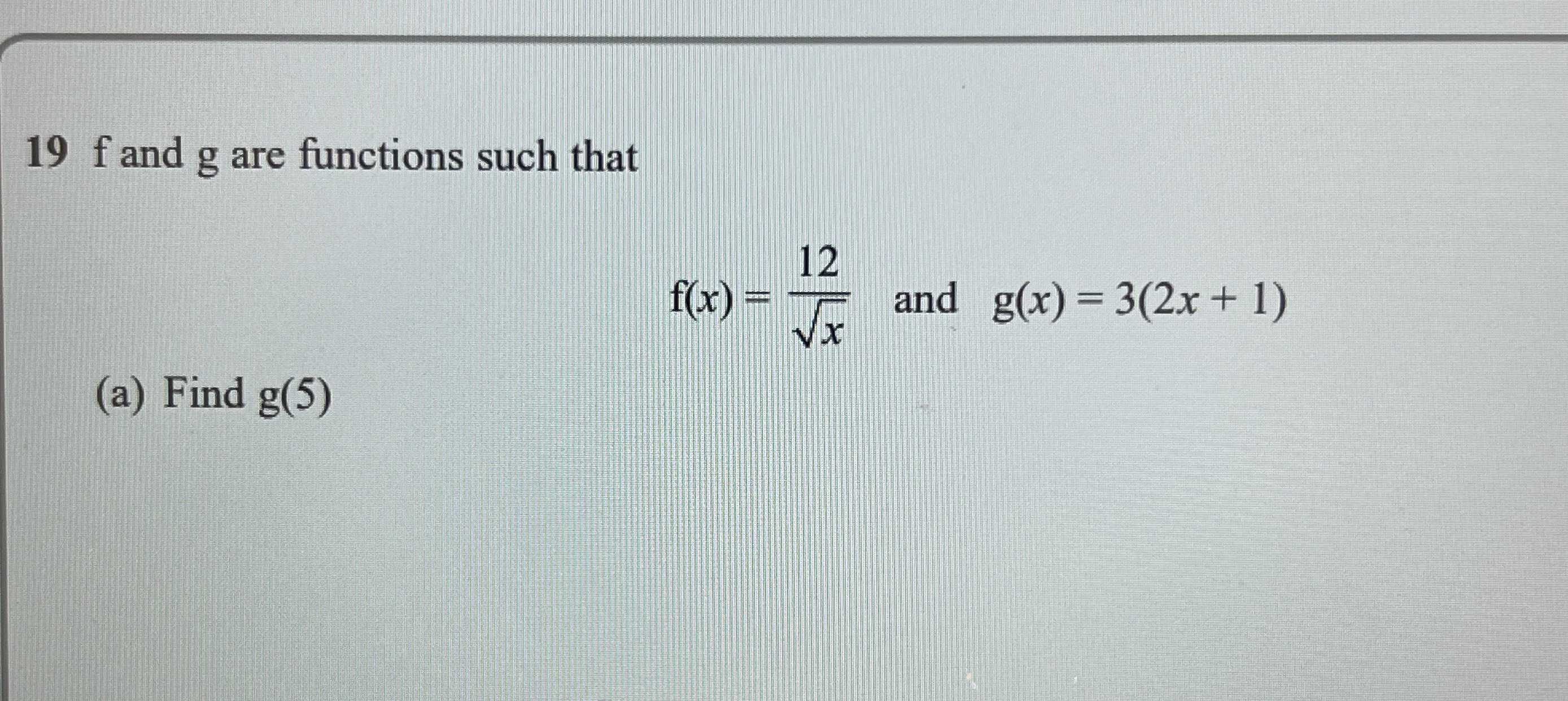### ¿Todavía tienes preguntas de matemáticas?

Pregunte a nuestros tutores expertos
Algebra
Pregunta$$19$$  f and $$g$$ are functions such that

$$f ( x ) = \frac { 12 } { \sqrt { x } }$$ and $$g ( x ) = 3 ( 2 x + 1 )$$

(a) Find $$g ( 5 )$$

$$g(5)= 33$$